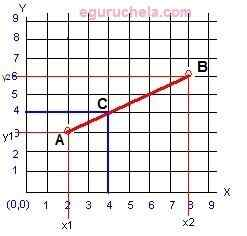# Linear Interpolation Value Calculator

Linear interpolation calculator finds y-axis value for given (x1 , y1) (x2 , y2) coordinates and targeted x-axis value.

Coordinatesxy
First (x1 , x1) :
Second (x2 , x2) :

 Target x :

 Interpolated y value:

### Formula

The line passing through two points A(x1 , y1 ) and B(y1 , y1 ) in 2D Cartesian coordinate plane is given by the equation as follows:

$$y=\frac{y_B-y_A}{x_B-x_A}(x-x_A)+y_A ,$$

where,

(xA,yA) and (xB,yB) are point coordinates of A and B

y-Coordinate of Interpolated Point:

The targeted x-axis value (Point C), y-coordinate of the point C on the linear interpolant AB is determined by the following equation

\begin{align} y_C&=\frac{y_B(x_C-x_A)+y_A(x_B-x_C)}{x_B-x_A}\end{align}

where

xC is the point coordinate of C,

(xA,yA) and (xB,yB) are point coordinates of A and B.

Linear interpolation is a method of curve fitting using linear polynomials. It is useful in numerical analysis, computer graphics. For given the two (x and y coordinates) points, the line is the linear interpolant between the points. The value of y at given x may be found by linear interpolation.